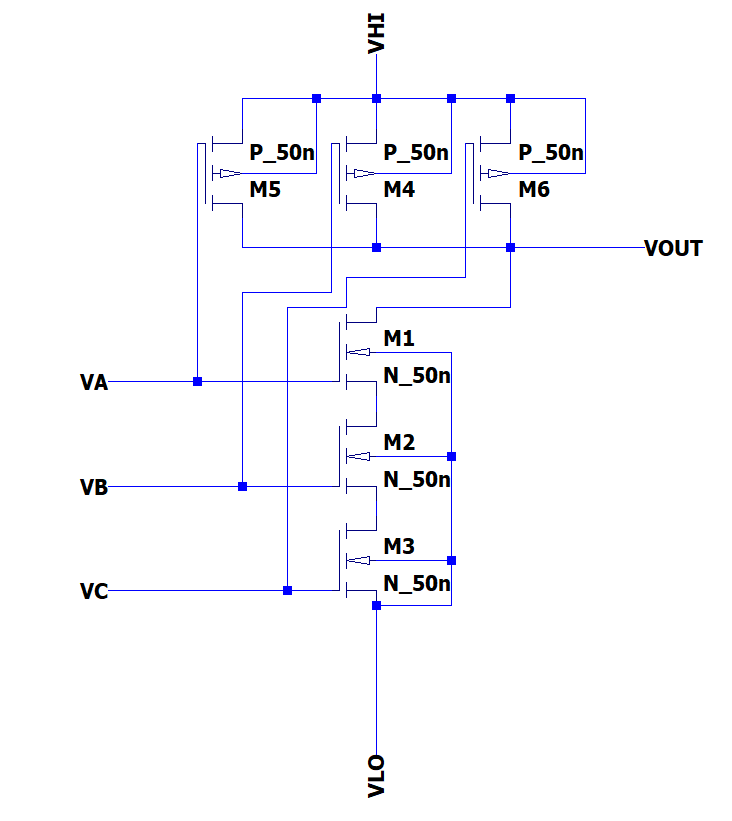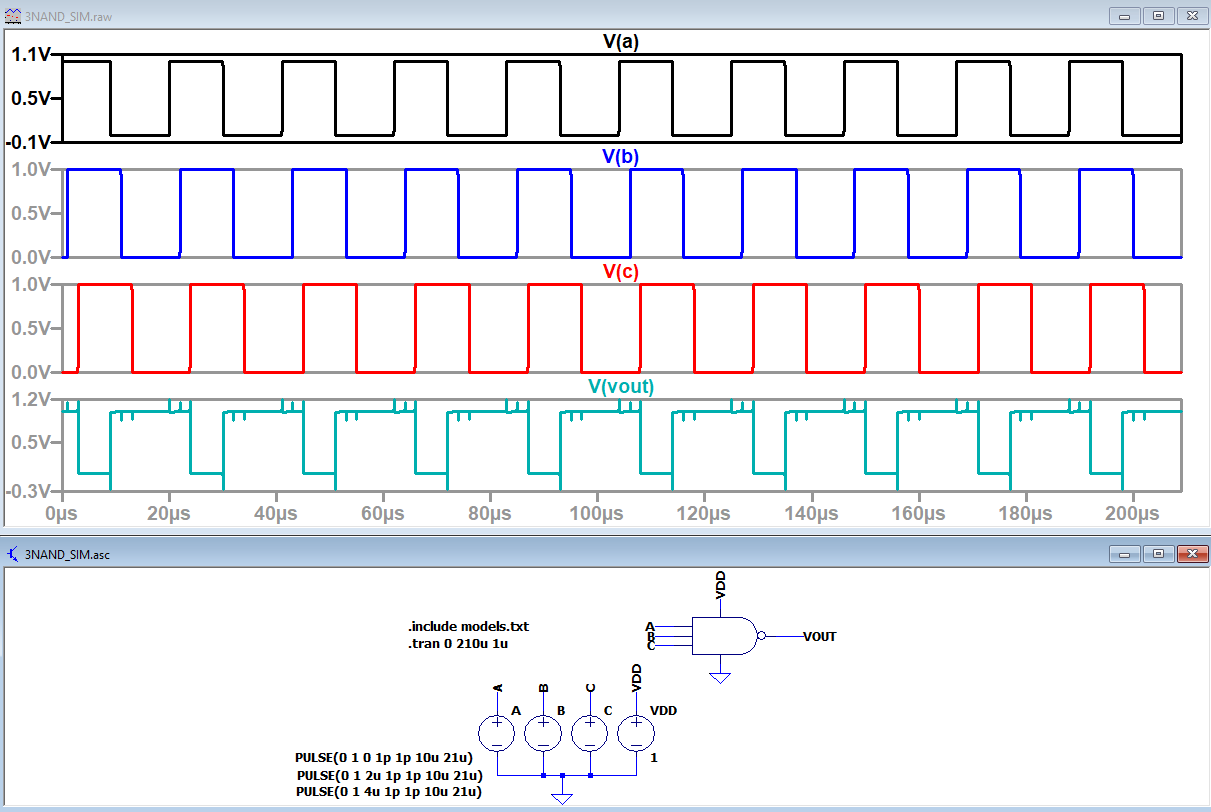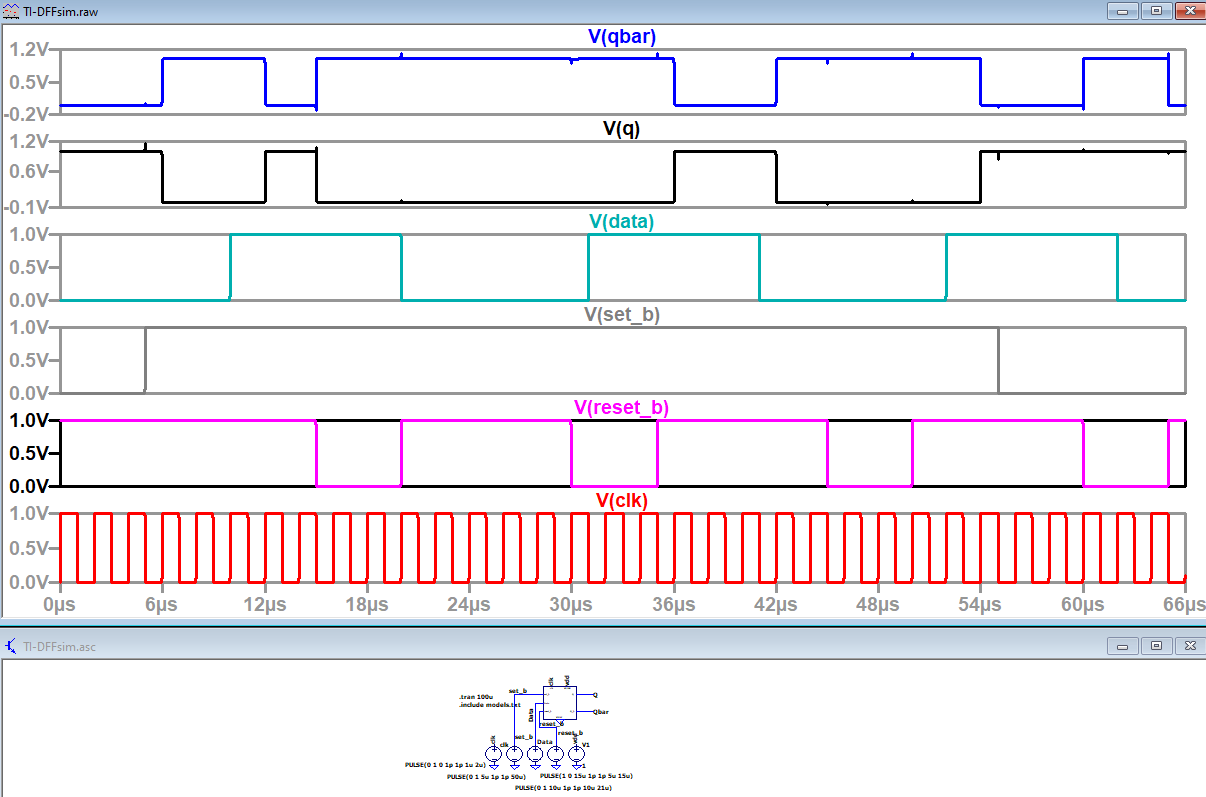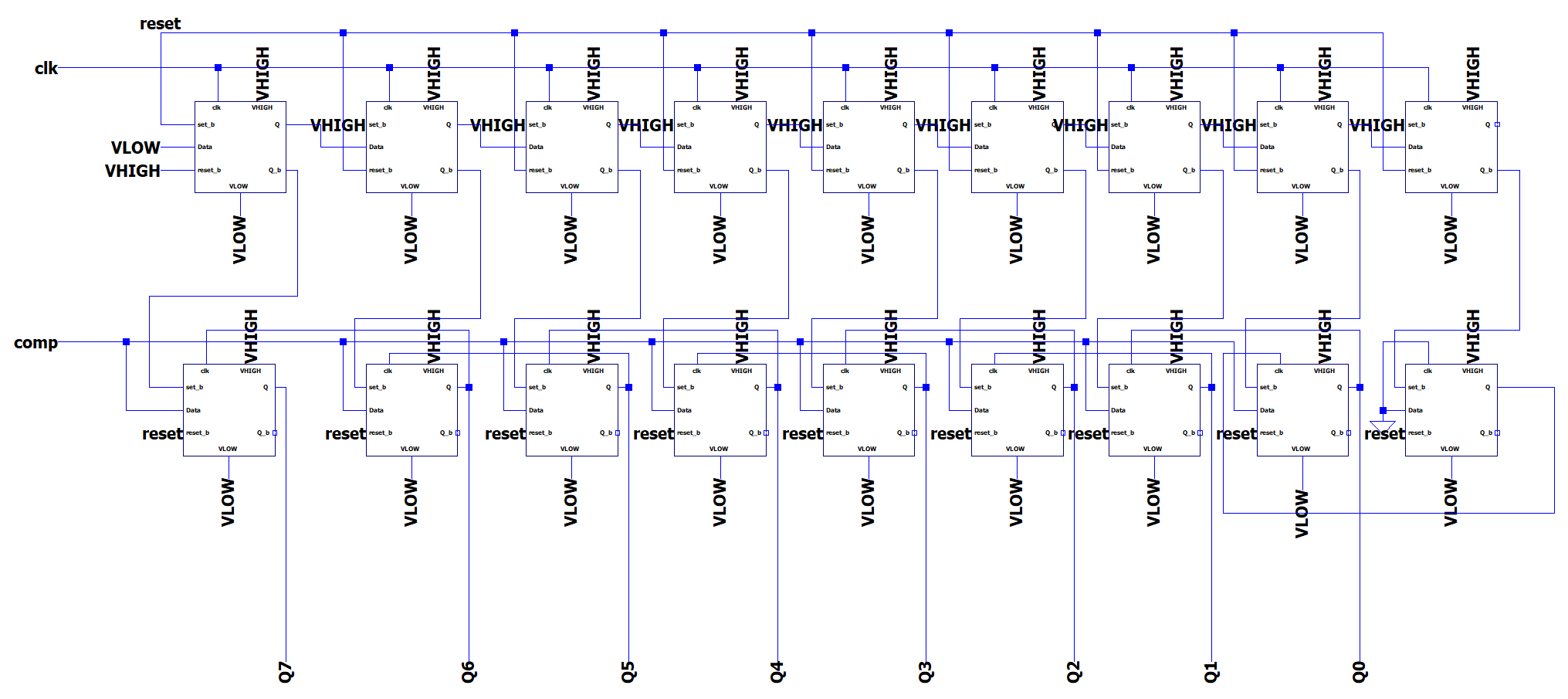ENGR338 Digital Electronics 2021 Spring
Final Project - Designing an 8-bit SAR ADC
Name: Ryan Jeanes

Email: rejeanes@fortlewis.edu

Introduction

Successive-Approximation Register Analog-to-Digital Converter (SAR ADC) is one of the types of ADCs that converts continuous analog waveforms into digital signal using a binary search through all possible outputs before settling on a digital output for each conversion operation. The SAR ADC is comprised of a sample and hold circuit, comparator, Digital to Analog Converter (DAC), and the SAR block. We are focusing on designing the SAR block itself, using 50nm NMOS and PMOS transistors so that they will operate at 1V logic instead of 5V logic.

Methods and Materials

We used LTSpice to create the schematics for the SAR block, the TI-DFF used in the SAR block, and the 3-input NAND gate used in the TI-DFF. After designing the schematics, all of the components were simulated to verify the logic.

Results

The logic for for the 3-input NAND was verified as shown in Figure 2, the DI-DFF was verified as shown in Figure 4, and the 8-bit SAR block logic was verified as shown in Figure 6. All components worked properly, although there was a bit of noise with each component.Figure 1 - Schematic of the 3-input NAND gate.Figure 2 - While there was a bit of noise around the state changes on the output, but it is not enough to be a problem.Figure 3 - Schematic of the TI-DFFFigure 4 - Simulation of the TI-DFF that shows the logic is working.Figure 5 - Schematic of the 8-bit SAR blockFigure 6 - Simulation of the 8-bit SAR block that shows the correct operations as well as the state changes being rising-edge triggered.

Discussion

The 8-bit SAR block functioned very well, and didn't have any noticeable noise like the 3-input NAND gate did. This SAR block being designed to work at 1V makes it a very useful ADC for low power systems, especially since it is stable at clock speeds higher than what was used to simulate it.